HOME plateWON | World!OfNumbersPalindromic Squares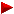FactorizationRecordsAll Squares up to length 31The Sporadic SquaresThe SubsetsExtra Squarestrianglepentahexaheptaoctanonan(n+1)n(n+2)n(n+x)n^2+1n^2+xn^2–xn^2+(n+1)n^2+(n+x)

Introduction

Palindromic numbers are numbers which read the same from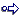left to right (forwards) as from the right to left (backwards)Here are a few random examples : 9, 9559, 4078704, 9876543210123456789

Square numbers are defined and calculated by this extraordinary intricate and excruciatingly complex formula.
So, this line is for experts onlyor ( base ) x ( base ) base 2
To the right a graphical representation of the first three square numbers to whet the beginner's appetite.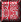square 1 square 4 square 9

 PLAIN TEXT SQUARES PLAIN TEXT SSP

Normal and Palindromic SquaresSo far this compilation counts 8028 square palindromic numbers.
Here is the largest Square Palindrome that Patrick De Geest
discovered, using CUDA code by Robert Xiao, on [ July 7, 2023 ].

 This basenumber 982.503.990.036.767.976.718.486.749.830.913has 33 digits yielding the following palindromic square record number with even number of digits 965.314.090.438.169.467.661.632.561.053.978.879.350.165.236.166.764.961.834.090.413.569 having a staggering length of 66 digits.

 This world record was achieved using CUDA code written by Robert Xiao and no longer on Rust. Recently he generalized the program to handle arbitrary quadratics. CUDA is a programming language, or more properly a programming toolkit, for writing software to run on GPUs rather than CPUs. It runs about 50 times faster on our GPUs though the logic of the code follows the Rust version closely. I asked Robert now that his CUDA is running at warp speed how far it would reach. He answered that as for 70 digits the time estimate on that is around ~400 days on one of our GPUs. 60 digits is about two days of GPU time, and it’ll go up by a factor of 10 every 4 digits. Doable but it’ll be a pretty decent power bill :) “Maybe we could get some palindrome enthusiasts together”, as David Griffeath put it, “and get a distributed computation going.” The program is very amenable to divide-and-conquer approaches.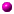All palindromic square numbers can only start or end with digits 0, 1, 4, 5, 6 or 9.
Alas, my palindromes may not have leading 0's! So the zero option must not be investigated.
1 can only be followed by an even number : 10, 12, 14, 16 or 18
4 can only be followed by an even number : 40, 42, 44, 46 or 48
5 can only be followed by 2 : 52
6 can only be followed by an odd number : 61, 63, 65, 67 or 69
9 can only be followed by an even number : 90, 92, 94, 96 or 98There exist no palindromic squares of length  2, 4, 8, 10, 14, 18, 20, 24, 30, 38, 40, 46, 54, 56, 62, 64.
(Sloane's A034822)

 Case Square Change of variables CUDApalin parameters Base Correction base ver 1 n.a. `A B C →  1 0 0 ` base = CUDAbase base ver 2 n = m + 1 `A B C →  1 2 1 ` base = CUDAbase + 1Mike Keith made an original study of the palindromic squares.
He was able to classify and enumerate them in a logical manner
and went even further as he tried also to create a general formula for
calculating the number of certain classes of palindromic squares,
except for the 'sporadic' solutions. Wonderful!
[Please refer to the “JRM” source detailed in section 'Sources Revealed'].

• The trivial (Tr) Solutions - ex. 3 - the single digits.
• The Four Families
1. The Binary Root (B) Family - ex. 10010001110001001 - only with digits 0 and 1.
2. The Ternary Root (T) Family - ex. 100000020000001 - only with digits 0, 1, 2 and always odd.
3. The Even Root (E) Family - ex. 2000001000002 - only with digits (0,2) or (0,1,2) and always even.
4. The Asymmetric Root (A) Family - ex. 10109901101 or 10110n01101 - the pseudopalindromes.
• Sporadic Solutions - ex. 83163115486 - THE MOST INTERESTING ONES paradoxically!

Mike had most trouble with the Asymmetric Root Family but thanks to
Dave Wilson's “lemma” he made significant progress.

 What are the conditions for creating a pseudopalindrome with a palindromic square ? Open this windowand read David W. Wilson's answer.Unlike Palindromic Triangulars where it is impossible to predict a next higher one, whether its basenumber is palindromic or not,
with the Palindromic Squares (and Cubes) we have an opposite situation. Finding a next higher number is very easy.
Start e.g. with the number 11. Then repeatedly add a zero between the two 'ones' and square them.
A pattern emerges that can go on forever.

BaseSquare
11121
10110201
10011002001
10001  100020001

There are other numbers with the same properties (e.g. 10101). The repunit numbers like '111' looks also a good candidate
for finding palindromic squares (not for cubes though) but the expansion doesn't go on forever. Nine 'ones' still produce
a palindrome when squared, but not ten 'ones'. It's a near miss as only the number 8 doesn't show up in the left part of the square.
This anomaly stays with longer repunits as well, so we must abandon this candidate.
If we take resort to higher basenumbers only then we can extend the pattern (for a while)!

BaseSquare
11
11121
11112321
11111234321
11111123454321
11111112345654321
11111111234567654321
11111111123456787654321
11111111112345678987654321
1111111111  1234567900987654321 = NOT palindromic in base 10 !

Here is how Natalie Dickendasher [ November 4, 2005 ] (email) figures them out quickly in her head and impresses friends or boss :
The highest digit of the sequence will always be the quantity of 1's in the number your are squaring.
As an example lets start with my salary for next year (yah right): what is 111111 squared ?
EASY !! This number has a total of 6 digits. Therefore the highest number in the palindrome will be 6
so count to 6 and then back down. The answer is 12345654321 .

Finding large palindromic squares is easy if you start with some palindromic basenumbers as explained above.
So, let's concentrate on the difficult ones namely those with a non palindromic basenumber. The first six are
(26 ) (264 ) (307 ) (836 ) (2285 ) and (2636 ) and can be found without too much trouble with a spreadsheet or calculator.

 Mike Bennett made an attempt in finding out what his chances are in discovering palindromic squares and palindromic cubes. Here is his mathematical analysis and the probability of findingthose interesting palindromic polygonal numbers.

Statistics with focus starting from Square Palindromes lengths

Square Palindrome(SP)_length 1 2 3 4 5 6 7 8 9 10 11 12 13 14 15 16 17 18 19 20 21 22 23 24 25 26 27 28 29 30 31 32 33 34 35 A263618 4 0 3 0 7 1 5 0 11 0 5 1 19 0 13 1 25 0 18 0 48 1 31 0 70 1 44 2 105 0 70 1 153 1 98 -- 0 1 0 2 1 2 0 3 0 0 1 5 0 4 1 1 0 2 0 6 1 4 0 4 1 1 2 3 0 5 1 5 1 4 -- 1 2 2 3 0 5 4 1 2 6 4 4 1 3 5 5 4 0 0 1 0 0 1 0 1 0 0 1 0 1 2 0 1 1 A263618 3 209 0 132 0 291 1 181 1 384 0 234 2 496 1 301 1 636 0 383 0 798 1 474 1 981 0 577 0 1196 2 - - - - 3 2 0 1 0 4 1 4 1 4 0 1 2 2 1 1 1 5 0 4 0 2 1 3 1 6 0 1 0 3 2 - - - - 2 1 4 4 4 1 2 1 5 4 2 3 6 1 3 - - 3 0 0 1 1 0 2 1 1 0 0 1 1 0 0 2 - -

Statistics with focus starting from Basenumber lengths

Basenumber(BN)_length 1 2 3 4 5 6 7 8 9 10 11 12 13 14 15 16 17 18 19 20 21 22 23 24 25 26 27 28 29 30 31 32 33 34 A263616 | A263617 4 3 8 5 11 6 19 14 25 18 49 31 71 46 105 71 154 101 209 132 292 182 384 236 497 302 636 383 799 475 981 578 1201 - -- 3 7 5 11 5 19 13 25 18 48 31 70 44 105 70 153 98 209 132 291 181 384 234 496 301 636 383 798 474 981 578 1199 - -- 0 1 0 0 1 0 1 0 0 1 0 1 2 0 1 1 3 0 0 1 1 0 2 1 1 0 0 1 1 0 0 2 - -- 1 3 2 3 1 5 5 1 2 7 4 5 3 3 6 6 7 2 1 5 5 4 3 3 2 5 4 3 4 6 1 5 - -- 1 2 2 3 0 5 4 1 2 6 4 4 1 3 5 5 4 2 1 4 4 4 1 2 1 5 4 2 3 6 1 3 - -- 2 5 3 8 5 14 9 24 16 42 27 66 43 102 65 148 94 207 131 287 177 380 233 494 300 631 379 796 471 975 577 1196 - A263614 -- 1 2 2 4 4 8 8 16 15 30 26 52 42 84 64 128 93 186 130 260 176 352 232 454 299 598 378 756 470 940 576 1152 - A142150 -- 0 1 0 2 0 3 0 4 0 5 0 6 0 7 0 8 0 9 0 10 0 11 0 12 0 13 0 14 0 15 0 16 - A007573 -- 0 0 0 0 0 1 0 2 0 5 0 6 0 9 0 10 0 10 0 15 0 15 0 16 0 18 0 24 0 18 0 26 - A000034 -- 1 2 1 2 1 2 1 2 1 2 1 2 1 2 1 2 1 2 1 2 1 2 1 2 1 2 1 2 1 2 1 2 -

Can we find formulas for all or some of these sequences ?

 Mike Keith gave two formulas in his article 'Classification and enumeration of palindromic squares' published in the “Journal of Recreational Mathematics” Volume 22, Number 2 - 1990, pp. 124-132. One for the Binary Root Family defined for all even N ⩾ 2 and another for the Binary & Ternary Root Family combined, defined for all odd N ⩾ 3. This is a choice that Keith made as it suited more in his scheme of things. Keith_|B| = (N3 – 6*N2 + 32*N) / 48 → for even values of N ⩾ 2 → bnl = N = 30 gives result  470  Keith_|B|+|T| = (N3 – 9*N2 + 59*N – 51) / 24→ for odd values of N ⩾ 3 → bnl = N = 31 gives result  955  ( = 940 + 15) Through David Griffeath we have now also the formula for odd values of N with the |B| separate from the |T|. Griffeath_|B| = (N3 – 9*N2 + 47*N – 39) / 24→ for odd values of N ⩾ 3 → bnl = N = 31 gives result  940  The total number of the Even Root family is simply |E| = 1 if N is even |E| = 2 if N is odd Regarding the Asymmetric family Keith writes “Finding an exact formula for |A| remains an open problem.” David Griffeath [ email from December 1, 2022 ] derives the right formulas for the # bnl binaries → |B|. “Start with a binary palindrome root of size 2n. It needs to have 1s on the ends. There are n–1 remaining spots on the right half where we can choose to put 0,1,2, or 3 additional 1s. Then symmetric spots must be chosen on the left side. So the total number of ways we can do this is C(n-1,0)+C(n-1,1) +C(n-1,2)+C(n-1,3). After some algebra this reduces to n(n^2 - 3n + 8)/6. Now suppose the size is 2n+1. Then any pattern of the type just described works for the digits to the right and left of the central digit, and that can be either 0 or 1. Thus the formula for 2n+1 is simply n(n^2 - 3n + 8)/3.” Griffeath_|B| = n*(n2 – 3*n + 8) / 6 → for even values of N → bnl = 30 (2n) so n=15 gives result  470  Griffeath_|B| = n*(n2 – 3*n + 8) / 3 → for odd values of N → bnl = 31 (2n+1) so n=15 gives result  940  D. Griffeath continues his mail with “As we know, whether there are an even or odd number of digits in a square palindrome root matters substantially. So it is natural to break up the formula this way. It is artificial to force these cases into a single formula. But this can be done by manipulating the coefficients of the two cubics above and adding a multiplier (-1)^n to one of the terms in order to account for parity. This is the essence of what is going on in a more complicated formula. I can go into more details if you like, but nothing illuminating is accomplished.” And yet such a single formula for all even/odd values of N in the |B| family can be found in OEIS A263614 and was added by Colin Barker. I'll give here his Pari/gp function (Barker_|B|) gp > a(n) = (-((-1)^n*(-78+62*n-12*n^2+n^3))+3*(-26+42*n-8*n^2+n^3))/96 After giving this command just type a(30) or a(31) to get  470  and  940  resp. It looks intriguingly complex especially compared with the easyness of programming these Binary Root solutions but less so when we start to dissect it... (   –((–1)n*(n3–12*n2+62*n–78)) + 3*(n3–8*n2+42*n–26)   ) / 96 And again David comes to the rescue and helps us unraveling the Barker formula. See panel on the right ↗
I've been feeling remiss about not showing you how that messy formula for the number of binaries doesn't
involve fancy math, but rather just a bunch of unpleasant, unenlightening algebra. So I've attached a
handwritten sketch of the computations.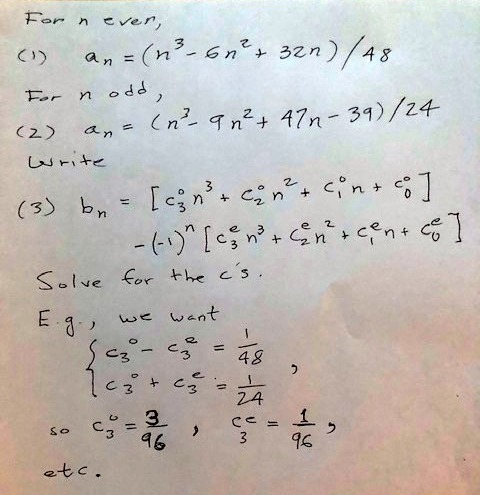The first step, in (1) and (2) is to change variables and express the formulas for the even and odd cases
in terms of the length. Just yucky algebra. Then to get the unified formula, we write the number of binaries
b_n as the sum of two cubics, one with the magic multiplier (-1)^n to flip between 1 and -1 in the even and
odd cases. The combination needs to flip between (1) and (2) as desired, so we solve for all the c's. I just
did the computation of the cubic power coefficients, but the rest are analogous. Just more yucky algebra.

Thanks David for this proper answer, much appreciated!"

Sources Revealed

 Neil Sloane's “Integer Sequences” Encyclopedia can be consulted online : Neil Sloane's Integer Sequences The normal squares are categorised as follows : %N The squares: a(n) = n^2. under A000290 The palindromic squares are categorised in the following manner : %N Square is a palindrome. under A002778 %N Palindromic Squares. under A002779. %N Numbers n such that n^2 is a palindromic square of sporadic type. under A059744 %N Palindromic squares of sporadic type. under A059745. Click here to view some of the author's [P. De Geest] entries to the table. Click here to view some entries to the table about palindromes.

An alternative way to get a 'structural' insight as how to imagine squares is to follow these sites :

Squares Acquaint yourself with SQUARES - by Richard Phillips From Eric Weisstein's Math Encyclopedia Someone gave me different numbers to find the square root of, including 14641.My answer was 121. Something special about this is that both numbers are readthe same left to right as right to left. Do you know any other numbers in whichboth the number and its square root are this way ?

A very interesting webpage by William Rex Marshall (1930-2010) is titled “Palindromic Squares”
and gives an overview of the first 67 palindromic squares known up to May 28, 2001.
The classification of these squares as set out by Michael Keith in the JRM from 1990 is also revealed.
http://www.geocities.com/williamrexmarshall/math/palsq.html (archived)

The second highest one (3069306930693 ) is copied from Martin Gardner's book “The Ambidextrous Universe”
see page 40.

Another source “Curious and Interesting Numbers” by David Wells, page 185, provided me the basenumber 798644 .

While surfing the Internet I came across the work of Keith Devlin namely All the Math that's Fit to Print.
In chapters 17, 75 and 110 he printed some palindromic squares he got from other readers.
The highest one that originates from his publication is 6360832925898 .

Gustavus J. Simmons published an article about palindromic squares in the “Journal of Recreational Mathematics”,
J. Rec. Math., 3 (No. 2, 1970), 93-98, titled “Palindromic Powers”.
OEIS Source (annotated scanned copy) → https://oeis.org/A002778/a002778_2.pdf

Gustavus J. Simmons published an article about palindromic squares in the “Journal of Recreational Mathematics”,
Volume 5, No. 1, 1972, pp. 11-19, titled “On Palindromic Squares of Non-Palindromic Numbers”.
OEIS Source (annotated scanned copy) → https://oeis.org/A002778/a002778.pdf

Mike Keith (email) (website) published an article about palindromic squares in the “Journal of Recreational Mathematics”,
Volume 22, Number 2 - 1990, pp. 124-132, titled “Classification and enumeration of palindromic squares”,
and was followed by an article by Charles Ashbacher, pp. 133-135, “More on palindromic squares”.
OEIS Source (annotated scanned copy) → https://oeis.org/A002778/a002778_1.pdf

I found Feng Yuan's record palindromic square of 55 digits  in the following archived blog
http://blogs.msdn.com/fyuan/archive/2008/01/31/sts-a-palindromic-word-i-will-remember.aspxClick here to displaythe full listing up to length 31.Click here to displaythe sporadics listing of all such square palindromes (SSP).Click here to displaythe subsets of palindromic squares.

Asymmetric (a.k.a. Pseudopalindromic or Core) Basenumbers

Careful observation of some numbers in the list lead me to the discovery of ever greater non-palindromic basenumbers whose squares are palindromic.
Specially the numbers with indexes , ,  and  attracted my attention because of the CORE number 091 in them.

 1109111
 110091011
 111091111
 11000910011
To the left there was the 'border' number 11 followed by 0, 1 and 2 zero's. To the right there was its reversal.
So without the core 091 we would have a palindromic basenumber but with 091 in the middle we always have
a non-palindromic basenumber ! The general layout becomes as follows :
11 + X_zero's + 091 + X_zero's + 11
No matter what value of X we always create a palindromic square.
The same is true if we substitute 11 with 111
111 + X_zero's + 091 + X_zero's + 111
I'm still investigating border values other then 11 or 111.
Borders like 1011 and 1101 also look promising...

A second promising CORE number exists. It is 09901 and it shows up from , , , ....

 10109901101
 1010099010101
 1011099011101
 100110990111001
 101000990100101
 101010990110101
 10010109901101001
 10100009901000101
 10100109901100101
 10101009901010101
As for now, I can't discover a regularity in it... Can you ?
It looks that this CORE number likes to be surrounded by 101 and the number zero.
Yes, it gives us a method to produce infinite palindromic squares once more :
101 + X_zero's + 09901 + X_zero's + 101

I think a new third CORE number is emerging... It is 0999001
Watch out from

 100109990011001
 10010099900101001
 10011099900111001

Quite interesting. Let's put these three CORE numbers in a row :

091
09901
0999001
Extending the pattern to the next one gives this CORE number of 9 digits :
099990001
We also observed that the length of the CORE basenumbers increases with 2 and so their respective squarelengths with 4.
As the table already shows all the CORE numbers up to length 33, we must at least add 5 digits on both sides to start with
basenumbers of lengths 19. Let's try some combinations :
1000009999000100001 yields no palindromic square but
1000109999000110001  does yield a palindromic square !
Just by playing around with beautiful patterns we reveal more palindromic squares.
Wonderful !

David W. Wilson took up the thread where I left off.
[ February 16, 1998 ].

If we allow a digit "n" with value "–1", then 091 is equal to the palindrome 1n1,
while 09901 = 10n01, 0999001 = 100n001, etc. Thus

10109901101 = 10110n01101 = 
is a sort of 'pseudopalindrome'. When we square it, lo and behold
```
10110n01101
x 10110n01101
-----------
10110n01101
10110n01101..
10110n01101...
n0nn010nn0n.....
10110n01101.......
10110n01101........
10110n01101..........
---------------------
102210100272001012201
```
we obtain a palindrome because the sums of the individual rows happen to lie between 0 and 9 inclusive.
Here's a palindromic square family :
111n1112 = 11091112 = 1230127210321 = 
101010n0101012 = 10100990101012 = 1020300010207020100030201 = 
100100100n0010010012 = 10010009990010010012 = 1002003000001002007002001000003002001 = 
etc.
I think you get the picture. The family is infinite, as is the family
1111n11112 = 
10101010n010101012 = 
etc.
In fact, any pseudopalindrome with a palindromic square can be interspersed with equal numbers of zeroes
to generate another pseudopalindrome with a palindromic square.

More Patterns

and that is finalized alternatively with a chopped off and rounded version of it namely a 3 or 307...

 3
 307
 3069 3
 3069 307
 3069 3069 3
 3069 3069 307
 3069 3069 3069 3
Each time the length increases with 2
and the length of the resulting squares increases with 4.
So, logically the next one should be 306930693069307 but alas... the square is NOT palindromic !
Oh well, it can not work every time ! On [ January 5, 1997 ] this near miss popped up on my
computerscreen : 306950094269977057. The system surely knows how to comfort me...

3069 occurs as displacements to powers of ten so that this number forms the nearest (probable) prime to those axes :

1010013069 is the largest prime of 1001 digits

 101001 – 3069 105068 – 3069 107565 – 3069 1019217 – 3069 1028898 – 3069 101555 + 3069 102399 + 3069 105016 + 3069 105063 + 3069 109896 + 3069

NEVER ODD OR EVENRead title backwards please !

It looks as though the decent sufficiently-random nonpatternlike unpredictable nonpalindromic basenumbers become very rare.
In the table (see Full Listing or the Subsets) I highlighted them using a lightblue background in the side-cells.
And even then a further distinction can be made. Consider the even and odd length values for a minute.
Sporadic Palindromic Squares with an even length are just a plain minority. Until now I can only list twenty of them.
These are highlighted in the table by means of a aquamarine background color in the side-cells.

 Sporadic Palindromic Squares of EVEN length
Score 'subset E' = 20

I 'almost' solved a very ancient problem namely : squaring the circle (pi = 3,1415....)
MORELAND PI welcomes PALINDROMES

    314155324482867

This basenumber starts with the the number 2201 which is (to me) the only known nonpalindromic basenumber
of a palindromic cube ! The cube of 2201 equalling 10662526601.

    2201019508986478

Here's another attractive but finite expansion starts with the number 1 whereby 01 is repeatedly added.
The following series arises :
BaseSquare
11
10110201
10101102030201
10101011020304030201
10101010110203040504030201
10101010101102030405060504030201
10101010101011020304050607060504030201
10101010101010110203040506070807060504030201
10101010101010101102030405060708090807060504030201 (° see conjecture below)
1010101010101010101  10203040506070809100908070605004030201 = QUITE UNpalindromic !

CONFIRMATION
10101010101010101 is indeed the smallest integer that generates
a palindromic square 102030405060708090807060504030201
that is also pandigital (contains all the ten digits).

Here is a good place to talk about a famous conjecture that nobody
could prove or disprove for more than 80 years.
I must thank Mike Keith for letting me know this fact through an email message.
Mike has heard that this conjecture originates from
L. E. Dickson's famous “History of the Theory of Numbers”.

History :
My table lists exhaustively all palindromic squares up to lengths 31.
I also listed all the palindromic squares of length 32.
Palindromic squares of  'even' nature seem to be very popular among the record
breaking submitters. They told me there are no gaps for the 'even' length 32 !
So, in fact I had to finish checking only length 33...
Of which I checked all squares beginning with 1, 5 and 9 !
( and 50 % of those beginning with digits 4 and 6 ).
I collected a total of 151 palindromic squares beginning with digit 1.
(Un)lucky for me Dickson's number 102030405060708090807060504030201
( 101010101010101012 )  is the 86th one. Anyway after checking these 86 squares
I could proudly announce that the conjecture was finally proven [ May 3, 1998 ].
The second smallest number  that answers to these rules is this one (also of length 33) :
118431915157648212 = 140261185279083838380972581162041.Sloane's A001110 gives the first numbers that are both Square and Triangular.
1, 36, 1225, 41616, 1413721, 48024900, ...
Consult also Eric Weisstein's page Square Triangular NumberSloane's A036353 gives the first numbers that are both Square and Pentagonal.
1, 9801, 94109401, 903638458801, 8676736387298001, ...
Consult also Eric Weisstein's page Square Pentagonal Number.Sloane's A036354 gives the first numbers that are both Square and Heptagonal.
1, 81, 5929, 2307361, 168662169, 12328771225, 4797839017609, ...
Consult also Eric Weisstein's page Square Heptagonal Number.Sloane's A036428 gives the first numbers that are both Square and Octagonal.
1, 225, 43681, 8473921, 1643897025, ...
Consult also Eric Weisstein's page Square Octagonal Number.Sloane's A036411 gives the first numbers that are both Square and Nonagonal.
1, 9, 1089, 8281, 978121, 7436529, ...
Consult also Eric Weisstein's page Square Nonagonal Number.

Alain Bex (email) gave also some palindromic squares in base 12.
Link to Palindromic Numbers other than Base 10

```

```

[TOP OF PAGE]

Patrick De Geest - Belgium- Short Bio - Some Pictures